# Electronics and Communication Engineering - Exam Questions Papers

11.

The amplitude of a random signal uniformly distributed between - 5 V and 5 V. If the positive values of the signal are uniformly quantized with a step size of 0.05 V, and the negative values are uniformly quantized with a step size of 0.10 V, the resulting signal to quantization noise is approximately

 A. 46 dB B. 43.8 dB C. 42 dB D. 40 dB

Explanation:

No answer description available for this question. Let us discuss.

12.

Consider the following statements:

1. With increase in temperature Fermi-level tries to come in between the energy gap exactly at middle
2. Mobility of electron in GaAs is very high compared to mobility of hole
3. In LED, the light energy radiated due to recombination with the aid of traps.
Which of these statements are true?

 A. 1 and 2 B. 1, 2 and 3 C. 2 and 3 D. 1 and 3

Explanation:

No answer description available for this question. Let us discuss.

13.

For good quality signal transmission all frequency components should have the same transmission delay, td and same phase shift-Φs. What can be said about the statement?

 A. Correct B. True from td but not for Φs C. Not true for td but true for Φs D. Both td and Φs are not involved in quality

Explanation:

No answer description available for this question. Let us discuss.

14.

The following series RLC circuit with zero initial conditions is excited by a unit impulse function δ(t)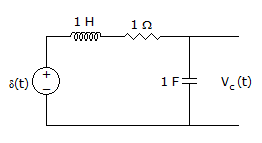For t > 0, the voltage across the resistor is

 A.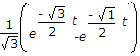B.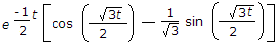C.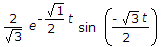D.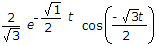Explanation:

No answer description available for this question. Let us discuss.

15.

In a CMOS CS amplifier, the active load is obtained by connecting a

 A. p channel current mirror circuit B. n channel transistor C. p channel transistor D. BJT current mirror